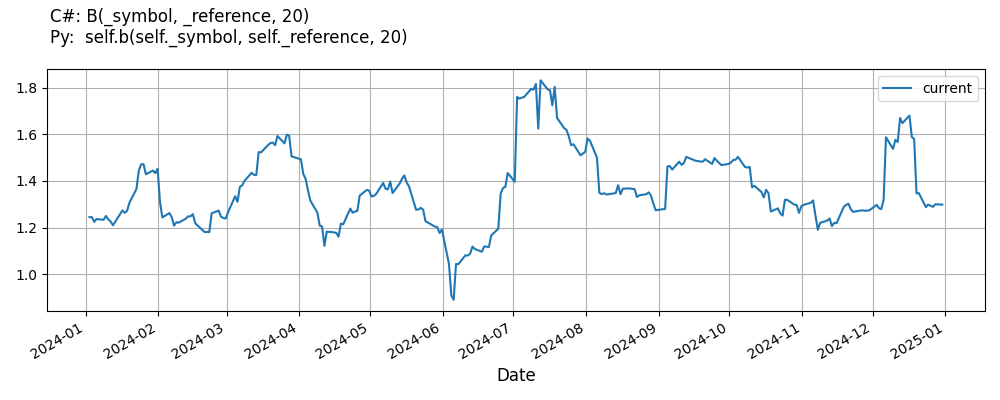# Supported Indicators

## Beta

### Introduction

In technical analysis Beta indicator is used to measure volatility or risk of a target (ETF) relative to the overall risk (volatility) of the reference (market indexes). The Beta indicators compares target's price movement to the movements of the indexes over the same period of time. It is common practice to use the SPX index as a benchmark of the overall reference market when it comes to Beta calculations.

### Using B Indicator

To create an automatic indicators for Beta, call the B helper method from the QCAlgorithm class. The B method creates a Beta object, hooks it up for automatic updates, and returns it so you can used it in your algorithm. In most cases, you should call the helper method in the Initialize method.

public class BetaAlgorithm : QCAlgorithm
{
private Symbol _symbol;
private Beta _b;

public override void Initialize()
{
_b = B("QQQ", "SPY", 20);
}

public override void OnData(Slice data)
{
{
Plot("Beta", "b", _b);
}
}
}
class BetaAlgorithm(QCAlgorithm):
def Initialize(self) -> None:
self.b = self.B("QQQ", "SPY", 20)

def OnData(self, slice: Slice) -> None:
self.Plot("Beta", "b", self.b.Current.Value)


The following reference table describes the B method:

### B()1/1

            Beta QuantConnect.Algorithm.QCAlgorithm.B (
Symbol                            target,
Symbol                            reference,
Int32                             period,
*Nullable<Resolution>       resolution,
*Func<IBaseData, TradeBar>  selector
)


Creates a Beta indicator for the given target symbol in relation with the reference used. The indicator will be automatically updated on the given resolution.

If you don't provide a resolution, it defaults to the security resolution. If you provide a resolution, it must be greater than or equal to the resolution of the security. For instance, if you subscribe to hourly data for a security, you should update its indicator with data that spans 1 hour or longer.

You can manually create a Beta indicator, so it doesn't automatically update. Manual indicators let you update their values with any data you choose.

Updating your indicator manually enables you to control when the indicator is updated and what data you use to update it. To manually update the indicator, call the Update method with a TradeBar. The indicator will only be ready after you prime it with enough data.

public class BetaAlgorithm : QCAlgorithm
{
private Symbol _symbol;
private Beta _b;

public override void Initialize()
{
_b = new Beta("SPY", 20);
}

public override void OnData(Slice data)
{
if (data.Bars.TryGeValue(_symbol, out var bar))
{
_b.Update(bar);
}

{
Plot("Beta", "b", _b);
}
}
}
class BetaAlgorithm(QCAlgorithm):
def Initialize(self) -> None:
self.b = Beta("SPY", 20)

def OnData(self, slice: Slice) -> None:
bar = data.Bars.get(self.symbol)
if bar:
self.b.Update(bar)

self.Plot("Beta", "b", self.b.Current.Value)


To register a manual indicator for automatic updates with the security data, call the RegisterIndicator method.

public class BetaAlgorithm : QCAlgorithm
{
private Symbol _symbol;
private Beta _b;

public override void Initialize()
{
_b = new Beta("SPY", 20);
RegisterIndicator(_symbol, _b, Resolution.Daily);
}

public override void OnData(Slice data)
{
{
Plot("Beta", "b", _b);
}
}
}
class BetaAlgorithm(QCAlgorithm):
def Initialize(self) -> None:
self.b = Beta("SPY", 20)
self.RegisterIndicator(self.symbol, self.b, Resolution.Daily)

def OnData(self, slice: Slice) -> None:
self.Plot("Beta", "b", self.b.Current.Value)


The following reference table describes the Beta constructor:

### Beta()1/1

            Beta QuantConnect.Indicators.Beta (
string  name,
int     period,
Symbol  targetSymbol,
Symbol  referenceSymbol
)


reference values.

### Visualization

The following image shows plot values of selected properties of Beta using the plotly library.You can also see our Videos. You can also get in touch with us via Discord.